## Organic Chemistry

Learn the toughest concepts covered in Organic Chemistry with step-by-step video tutorials and practice problems by world-class tutors.

1. A Review of General Chemistry

# Skeletal Structure

Since we are going to be drawing A LOT of molecules over the next year, it makes sense to find an easier way to draw them. Hence, I now present to you 🥁 the skeletal structure!

*Also known as bondline structure or line-angle structure.

Things You Don't Have to Draw
• Carbons are implied:Every corner is assumed to represent a carbon.
• Hydrogens are implied:Carbon is assumed to possess enough hydrogens to fill its octets.
• Lone Pairs are implied:Heteroatoms are assumed to possess enough electrons to fill their octets.
• Formal Charges are used to indicate when an atom does not satisfy its bonding preference.

Watch Out: ALL hydrogens on heteroatoms MUST be drawn explicitly.

1
concept

## How bondline is different from Lewis Structures.4m
Play a video:
So the bond line method is a way to simplify drawings of organic molecules based on the octet rule. So as long as you have a good understanding of the octet rule, you should be fine. Like you should do very well at be able to convert drawings into bond light. All right, so it turns out that in order to save time, we're gonna be implying a lot of different types of information that you can derive from the octave rule. So the first thing that we're gonna do is that remember that organic chemistry is the study of basically carbon and carbon structures. So if we have to write out every single carbon part of my friends, that's gonna be a bitch. Right? So what we want to do is figure out a way that we don't have to write C, C, c c like for 100 times in a row. So in the bottom line structures, car bombing structures, carbons are implied. Okay, So what that means is that every corner we're gonna have, like, a zigzag pattern and every corner is gonna be assumed to represent a carbon. I'll show you how that looks in a second. Another thing. That there's a lot of inorganic chemistry where we're trying to eliminate repetitive things. There's a lot of hydrogen. Okay, well, it turns out that hydrogen czar also gonna be implied. Why? Because it would suck to have to write all of them. All right, well, then, if they're not, if we're not drawing hydrants, how do we know how many there are? Well, all I have to do is use the octet rule. We said that every carbon is assumed to possess enough hydrogen to fill a talk Tet. So, for example, if a carbon has to bonds to other carbons and it doesn't, we don't show any hydrogen. We have toe add bonds there in order for that carbon to fulfill its octet. So think about it this way. Carbon oneself. How many electrons in its octet? Eight. If it only has two bonds to carbon, that means that there must be two bonds to hydrogen. That's the way we think. We kind of do a little bit of mental subtraction. And that's the number of hydrogen that are gonna be implied on that structure. Okay, so what else is implied? Lone pairs Because lone pairs would be super repetitive, too. Okay, lone pairs are implied. What that means is that, um we're not going to draw all the lone pairs. We're just gonna assume that hetero atoms will have enough lone pairs toe folder octet unless otherwise stated. Unless there's a charge. Okay. Now, by the way, I have to define something. What the heck is ahead? Or a lot of I just busted that out on you guys. A hetero atom is just any atom that is different from carbon. So I'm actually just going to say it's non carbon, non carbon atom. Okay, So what that means is that nitrogen, oxygen, flooring, all of those would be hetero atoms, and we're not gonna draw lone pairs. We're just gonna assume that they have enough lone pairs to fulfill their octet unless otherwise stated. What we use for that is formal charges. So now that we know how to do formal charges, formal charges are a huge part of the bond line structure. We never draw lone pairs. We only draw formal charges. And we use formal charges when Adam does not satisfy its bonding preference. So if there's a messed up bonding preference. We use a formal charge. We don't actually draw the lone pairs. Okay, There's one extra rule rule that you guys should be aware of that There's one tricky thing. All hydrogen on hetero atoms. Do you remember what a hetero atom once again? Non carton. So oxygen, whatever nitrogen all headings on hetero atoms must be drawn explicitly. Okay, The reason is because that could get tricky if we just don't draw the lone pairs. And if we also don't draw the hydrogen on a hetero atom, then I wouldn't know. Doesn't have a hydrogen. Doesn't have a lone pair, would get confusing. So in order to avoid that, we actually are going to include all the h Is that air drawn directly on a header? One. Okay.
2
example

## Conversion of ethanol from electron dot to bondline1m
Play a video:
so I wanted to show you guys this little diagram that I drew showing how we can go from one to the other. So the electron dot structure is like the most simple form of structure that we drew in. Gen. Cam, Basically what we what we had was that we drew out every loan, every electron explicitly, and we would show that every single bond was by used was made by the sharing of two electrons. Okay, then when we converted toe Lewis structure Lewis structure is also used in gen camps. You should be aware of it, but Lewis structure was just a little bit different. The only difference is that instead of showing the dots being shared, it replaced dots with sticks. So what it did was anytime they had dots being shared, it replaced them with sticks. And that was a Louis structure. But notice that a Lewis structures still kind of annoying because I have to draw every carbon, have to draw every hydrogen, and I have to draw every lone pair. So it's gonna take me a long time to draw everything in Lewis. So then we decide. Okay, Wolf, is there a faster way to do this. Well, bond line. Look how fast that is to draw because I'm ignoring the carbons. I'm just saying that every corner represents a carbon. Okay, I'm ignoring all of the hydrogen is attached to carbon. Because I'm just assuming that if this carbon has to bonds, that must mean that it has to hydrogen. And if this carbon has one bond attached to it, then it must have three hydrogen. Alright, then I'm implying the lone pairs and not drawing them, because I'm assuming that it has enough lone pairs to fulfill its octet. And then lastly, I am including that h because it would be confusing if I didn't include the h. All right, so that is the way bond line works. And that's what we're gonna do. Basically, for the rest of this topic, we're just gonna do a bunch of practice problems for this. So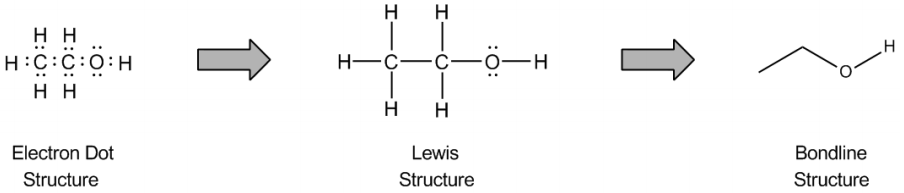3
Problem

How many implied hydrogens does each labeled carbon have?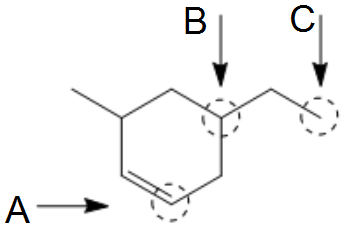4
Problem

How many implied hydrogens does each labeled carbon have?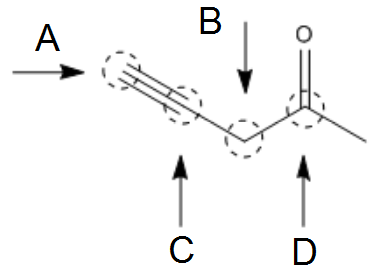5
Problem

Convert the structure into a line-angle structure. Be sure to assign ALL necessary formal and net charges.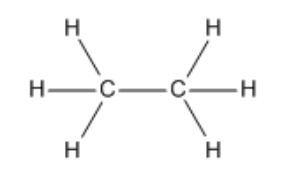6
Problem

Convert the structure into a line-angle structure. Be sure to assign ALL necessary formal and net charges.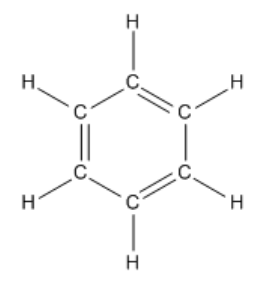7
Problem

Convert the structure into a line-angle structure. Be sure to assign ALL necessary formal and net charges.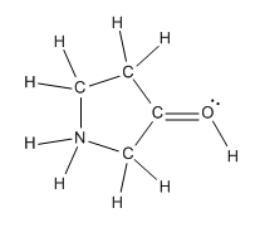Hint:Remember that you need to include formal charges if atoms are not at their bond preference.

8
Problem

Convert the structure into a line-angle structure. Be sure to assign ALL necessary formal and net charges.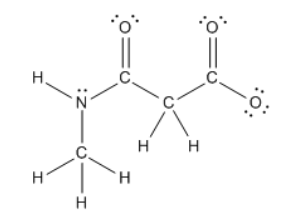We’ll be drawing these for the rest of the semester. Hope you liking them so far!